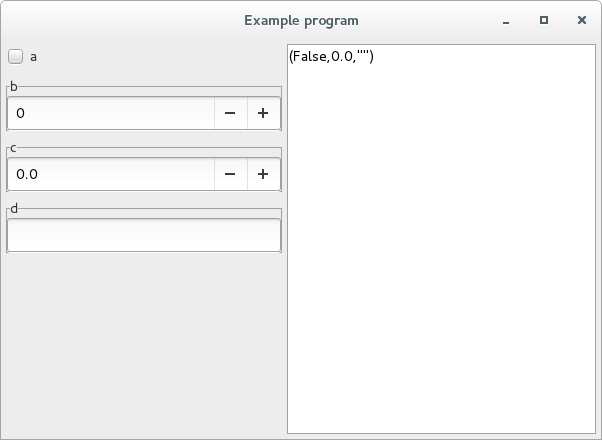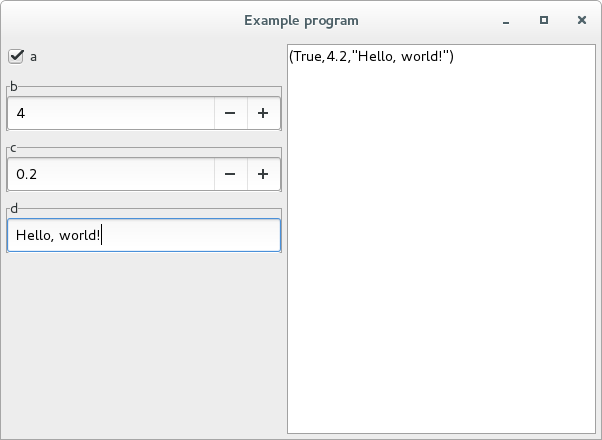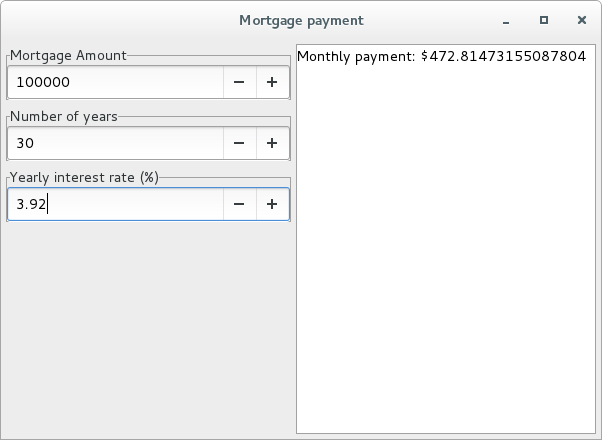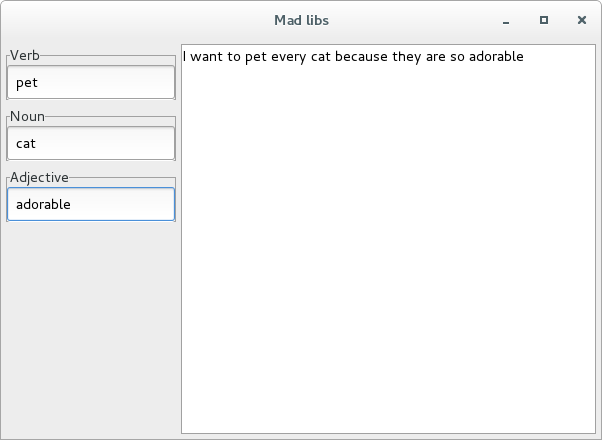## Wednesday, November 18, 2015

### Interactive and composable charts

I've added a `diagrams` backend to my `typed-spreadsheet` library which you can use to build composable graphical programs that update in response to user input.

Here's an example program that displays a circle that changes in response to various user inputs:

``````{-# LANGUAGE OverloadedStrings #-}

import Diagrams.Backend.Cairo (Cairo)
import Diagrams.Prelude

data AColor = Red | Orange | Yellow | Green | Blue | Purple
deriving (Enum, Bounded, Show)

toColor :: AColor -> Colour Double
toColor Red    = red
toColor Orange = orange
toColor Yellow = yellow
toColor Green  = green
toColor Blue   = blue
toColor Purple = purple

main :: IO ()
main = graphicalUI "Example program" logic
where
logic = combine <\$> radioButton      "Color"        Red [Orange .. Purple]
<*> spinButton       "X Coordinate" 1
<*> spinButton       "Y Coordinate" 1

combine :: AColor -> Double -> Double -> Double -> Diagram Cairo
combine color r x y =
circle r # fc (toColor color) # translate (r2 (x, -y))``````

Here is a video showing the example program in action:

#### Applicatives

The first half of the `main` function (named `logic`) requests four users inputs to parametrize the displayed circle:

• A radio button for selecting the circle's color
• A spin button for controlling radius which defaults to 100 (pixels)
• A spin button for controlling the x coordinate for the center of the circle
• A spin button for controlling the y coordinate for the center of the circle

Each of these inputs is an `Updatable` value and we can express that using Haskell's type system:

``````radioButton      "Color"        Red [Orange .. Purple] :: Updatable AColor
spinButtonAt 100 "Radius"       1                      :: Updatable Double
spinButton       "X Coordinate" 1                      :: Updatable Double
spinButton       "Y Coordinate" 1                      :: Updatable Double``````

The `Updatable` type implements Haskell's `Applicative` interface, meaning that you can combine smaller `Updatable` values into larger `Updatable` values using `Applicative` operations.

For example, consider this pure function that consumes four pure inputs and produces a pure diagram:

``````combine
:: AColor
-> Double
-> Double
-> Double
-> Diagram Cairo``````

Normally if we compute a pure function we would write something like this:

``````combine Green 40 10 20
:: Diagram Cairo``````

However, this result is static and unchanging. I would like to transform this function into one that accepts `Updatable` arguments and produces an `Updatable` result.

Fortunately, Haskell's `Applicative` interface lets us do just that. We can lift any pure function to operate on any type that implements the `Applicative` interface like the `Updatable` type. All we have to do is separate the function from the first argument using the `(<\$>)` operator and separate each subsequent argument using the `(<*>)` operator:

``````combine <\$> radioButton      "Color"        Red [Orange .. Purple]
<*> spinButton       "X Coordinate" 1
<*> spinButton       "Y Coordinate" 1
:: Updatable (Diagram Cairo)``````

Now the `combine` function accepts four `Updatable` arguments and produces an `Updatable` result! I can then pass this result to the `graphicalUI` function which builds a user interface from any `Updatable` `Diagram`:

``````graphicalUI :: Text -> Updatable Diagram -> IO ()

main = graphicalUI "Example program" logic``````

The `Applicative` operations ensure that every time one of our primitive `Updatable` inputs change, the composite `Updatable` `Diagram` immediately reflects that change.

#### Charts

One reason I wanted `diagrams` integration was to begin building interactive charts for typed spreadsheets. I'll illustrate this using a running example where I building up a successively more complex chart piece-by-piece.

Let's begin with a simple rectangle with an adjustable height (starting at 100 pixels):

``````{-# LANGUAGE OverloadedStrings #-}

import Diagrams.Backend.Cairo (Cairo)
import Diagrams.Prelude

import qualified Data.Text as Text

bar :: Int -> Updatable (Diagram Cairo)
bar i = fmap buildRect (spinButtonAt 100 label 1)
where
buildRect height = alignB (rect 30 height)

label = "Bar #" <> Text.pack (show i)

main :: IO ()
main = graphicalUI "Example program" (bar 1)``````

When we run this example we get a boring chart with a single bar:

However, the beauty of Haskell is composable abstractions like `Applicative`. We can take smaller pieces and very easily combine them into larger pieces. Each piece does one thing and does it well, and we compose them to build larger functionality from sound building blocks.

For example, if I want to create a bar chart with five individually updatable bars, I only need to add a few lines of code to create five bars and connect them:

``````{-# LANGUAGE OverloadedStrings #-}

import Diagrams.Backend.Cairo (Cairo)
import Diagrams.Prelude

import qualified Data.Text as Text

bar :: Int -> Updatable (Diagram Cairo)
bar i = fmap buildRect (spinButtonAt 100 label 1)
where
buildRect height = alignB (rect 30 height)

label = "Bar #" <> Text.pack (show i)

bars :: Int -> Updatable (Diagram Cairo)
bars n = fmap combine (traverse bar [1..n])
where
combine bars = alignX 0 (hcat bars)

main :: IO ()
main = graphicalUI "Example program" (bars 5)``````

This not only creates a bar chart with five bars, but also auto-generates a corresponding input cell for each bar:

Even better, all the inputs are strongly typed! The program enforces that all inputs are well-formed and won't let us input non-numeric values.

We also benefit from all the features of Haskell's `diagrams` library, which is an powerful Haskell library for building diagrams. Let's spruce up the diagram a little bit by adding color, spacing, and other embellishments:

``````{-# LANGUAGE FlexibleContexts  #-}
{-# LANGUAGE TypeFamilies      #-}

import Diagrams.Backend.Cairo (Cairo)
import Diagrams.Prelude

import qualified Data.Text as Text

bar :: Int -> Updatable (Diagram Cairo)
bar i = fmap buildBar (spinButtonAt 100 label 0.2)
where
color = case i `mod` 3 of
0 -> red
1 -> green
2 -> yellow

buildBar height =
(  alignB (   vine
<>  bubbles
)
<> alignB (   roundedRect 25 (height - 5) 5 # fc white
<>  roundedRect 30  height      5 # fc color
)
)
where
stepSize = 15

vine = strokeP (fromVertices (map toPoint [0..height]))
where
toPoint n = p2 (5 * cos (pi * n / stepSize), n)

bubble n =
# translate (r2 (0, n * stepSize))
# fc lightblue
where
radius = max 1 (min stepSize (height - n * stepSize)) / 5

bubbles = foldMap bubble [1 .. (height / stepSize) - 1]

label = "Bar #" <> Text.pack (show i)

bars :: Int -> Updatable (Diagram Cairo)
bars n = fmap combine (traverse bar [1..n])
where
combine bars = alignX 0 (hsep 5 [alignL yAxis, alignL (hsep 5 bars)])

yAxis = arrowV (r2 (0, 150))

main :: IO ()
main = graphicalUI "Example program" (bars 5)``````

One embellishment is a little animation where bubbles fade in and out near the top of the bar:

We can customize the visuals to our heart's content becuse the spreadsheet and diagram logic are both embedded within a fully featured programming language.

#### Conclusion

The `typed-spreadsheet` library illustrates how you can use the Haskell language to build high-level APIs that abstract way low-level details such as form building or reactive updates in this case.

In many languages high-level abstractions come at a price: you typically have to learn a domain-specific language in order to take advantage of high-level features. However, Haskell provides reusable interfaces like `Applicative`s that you learn once and apply over and over and over to each new library that you learn. This makes the Haskell learning curve very much like a "plateau": initially steep as you learn the reusable interfaces but then very flat as you repeatedly apply those interfaces in many diverse domains.

If you would like contribute to the `typed-spreadsheet` library you can contribute out-of-the-box charting functionality to help the library achieve feature parity with real spreadsheet software.

## Wednesday, November 11, 2015

I'm releasing the `typed-spreadsheet` library, which lets you build spreadsheets integrated with Haskell. I use the term "spreadsheet" a bit loosely, so I'll clarify what I mean by comparing and contrasting this library with traditional spreadsheets.

The best way to explain how this works is to begin with a small example:

``````{-# LANGUAGE OverloadedStrings #-}

import Control.Applicative

main :: IO ()
main = textUI "Example program" logic
where
-- Hate weird operators?  Read on!
logic = combine <\$> checkBox   "a"     -- Input #1
<*> spinButton "b" 1   -- Input #2
<*> spinButton "c" 0.1 -- Input #3
<*> entry      "d"     -- Input #4

combine a b c d = display (a, b + c, d) -- The output is a
-- function of all
-- four inputs``````

The above program builds a graphical user interface with four user inputs in the left panel and one output in the right panel:The output is a text representation of a 3-tuple whose:

• 1st element is the checkbox state (`False` for unchecked, `True` for checked)
• 2nd element is the sum of the two numeric fields (labeled "b" and "c")
• 3rd element is the text entry field

The right panel immediately updates in response to any user input from the left panel. For example, every time we toggle the checkbox or enter numbers/text the right panel changes:So in one sense this resembles a spreadsheet in that the output "cell" on the right (the text panel) updates immediately in response to the input "cell"s on the left (the checkbox, and numeric/text entry fields).

However, this also departs significantly from the traditional spreadsheet model: input controls reflect the type of input in order to make invalid inputs unrepresentable. For example, a `Bool` input corresponds to a checkbox.

#### Distribution

The generated executable is an ordinary binary so you can distribute the program to other users without needing to supply the Haskell compiler or toolchain. You can even fully statically link the executable for extra portability.

For example, say that I want to create a mortage calculator for somebody else to use. I can just write the following program:

``````{-# LANGUAGE OverloadedStrings #-}

import Control.Applicative
import Data.Monoid
import Data.Text (Text)

payment :: Double -> Double -> Double -> Text
payment mortgageAmount numberOfYears yearlyInterestRate
=  "Monthly payment: \$"
<> display (mortgageAmount * (i * (1 + i) ^ n) / ((1 + i) ^ n - 1))
where
n = truncate (numberOfYears * 12)
i = yearlyInterestRate / 12 / 100

logic :: Updatable Text
logic = payment <\$> spinButton "Mortgage Amount"          1000
<*> spinButton "Number of years"             1
<*> spinButton "Yearly interest rate (%)"    0.01

main :: IO ()
main = textUI "Mortgage payment" logic``````

... and compile that into an executable which I can give them. When they run the program they will get the following simple user interface:Or maybe I want to write a simple "mad libs" program for my son to play:

``````{-# LANGUAGE OverloadedStrings #-}

import Data.Monoid

noun = entry "Noun"

verb = entry "Verb"

example =
"I want to " <> verb <> " every " <> noun <> " because they are so " <> adjective

main :: IO ()
main = textUI "Mad libs" example``````

This generates the following interface:All the above examples have one thing in common: they only generate a single `Text` output. The `typed-spreadsheet` library does not permit multiple outputs or outputs other than `Text`. If we want to display multiple outputs then we need to somehow format and render all of them within a single `Text` value.

In the future the library may provide support for `diagrams` output instead of `Text` but for now I only provide `Text` outputs for simplicity.

#### Applicatives

The central type of this library is the `Updatable` type, which implements the `Applicative` interface. This interface lets us combine smaller `Updatable` values into larger `Updatable` values. For example, a `checkBox` takes a single `Text` argument (the label) and returns an `Updatable` `Bool`:

``checkBox :: Text -> Updatable Bool``

Using `Applicative` operators, `(<\$>)` and `(<*>)`, we can lift any function over an arbitrary number of `Updatable` values. For example, here is how I would lift the binary `(&&)` operator over two check boxes:

``````z :: Updatable Bool
z = (&&) <\$> checkBox "x" <*> checkBox "y"``````

... or combine their output into a tuple:

``````both :: Updatable (Bool, Bool)
both = (,) <\$> checkBox "x" <*> checkBox "y"``````

However, to the untrained eye these will look like operator soup. Heck, even to the trained eye they aren't that pretty (in my opinion).

Fortunately, `ghc-8.0` will come with a new `ApplicativeDo` which will greatly simplify programs that use the `Applicative` interface. For example, the above two examples would become much more readable:

``````z :: Updatable Bool
z = do
x <- checkBox "x"
y <- checkBox "y"
return (x && y)

both :: Updatable (Bool, Bool)
both = do
x <- checkBox "x"
y <- checkBox "y"
return (x, y)``````

Similarly, the very first example simplifies to:

``````{-# LANGUAGE ApplicativeDo     #-}

main :: IO ()
main = textUI "Example program" (do
a <- checkBox   "a"
b <- spinButton "b" 1
c <- spinButton "c" 0.1
d <- entry      "d"
return (display (a, b + c, d)) )``````

That's much easier on the eyes. `ApplicativeDo` helps the code look much less like operator soup and presents a comfortable syntax for people used to imperative programming.

#### Conclusion

• Adding new backends for output (like `diagrams`)